## Groups of Numbers

In doing basic math, you work with many different groups of numbers. The more you know about these groups, the easier they are to understand and work with.

• Natural or counting numbers: 1, 2, 3, 4, …
• Whole numbers: 0, 1, 2, 3, 4, …
• Integers: … –3, –2, –1, 0, 1, 2, 3, …
• Negative integers: … –3, –2, –1
• Positive integers: 1, 2, 3, … (the natural numbers)
Note: Zero is neither positive nor negative. It is neutral.
• Odd numbers; integers not divisible by 2:
• … – 5, – 3, – 1, 1, 3, 5, …
• Even numbers: Integers divisible by 2:
• … – 6, – 4, – 2, 2, 4, 6, …
• Rational numbers: Fractions, such as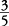or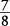. All integers are rational numbers; for example, the number 5 may be written as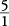. All rational numbers can be written as fractions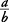, with a being an integer and b being a natural number. Both terminating decimals (such as 0.5) and repeating decimals (such as 0.333 … ) are also rational numbers because they can be written as fractions in this form.
• Irrational numbers: Numbers that cannot be written as fractions, with abeing an integer and b being a natural number.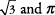(the Greek letter pi) are examples of irrational numbers.